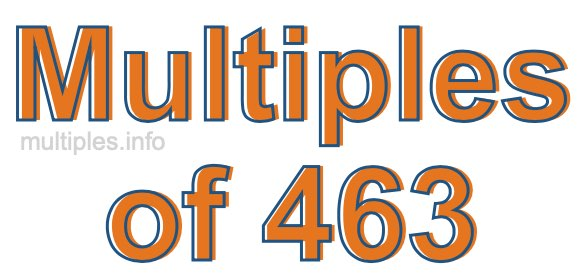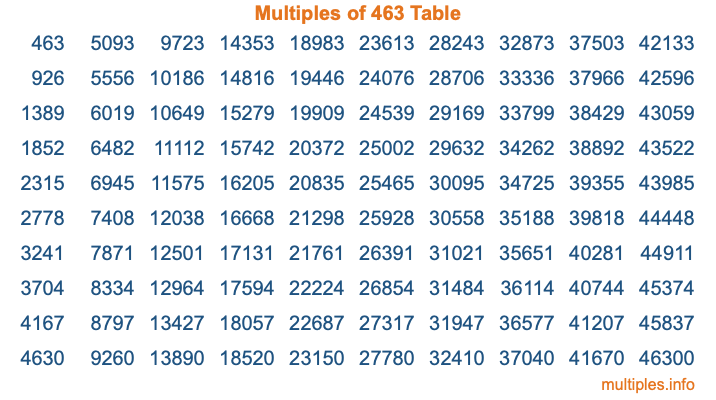Multiples of 463Welcome to the Multiples of 463 page. Here we will first teach you everything you will ever need to know about the multiples of 463, and then give you a study guide summary of everything we taught you to make sure you remember it all. Use this page to look up facts and learn information about the multiples of 463. This page will make you a multiples of four hundred sixty-three expert!

Definition of Multiples of 463
Multiples of 463 are all the numbers that when divided by 463 equal an integer. Each of the multiples of 463 are called a multiple. A multiple of 463 is created by multiplying 463 by an integer.

Therefore, to create a list of multiples of 463, you start with 1 multiplied by 463, then 2 multiplied by 463, then 3 multiplied by 463, and so on for as long as you want. Thus, the list of the first five multiples of 463 is 463, 926, 1389, 1852, and 2315. To see a larger list of multiples of 463, see the printable image of Multiples of 463 further down on this page. We also have a category where you can choose any nth multiple of 463.

Multiples of 463 Checker
The Multiples of 463 Checker below checks to see if any number of your choice is a multiple of 463. In other words, it checks to see if there is any number (integer) that when multiplied by 463 will equal your number. To do that, we divide your number by 463. If the the quotient is an integer, then your number is a multiple of 463.

Is  a multiple of 463?

Least Common Multiple of 463 and ...
A Least Common Multiple (LCM) is the lowest multiple that two or more numbers have in common. This is also called the smallest common multiple or lowest common multiple and is useful to know when you are adding our subtracting fractions. Enter one or more numbers below (463 is already entered) to find the LCM.

Check out our LCM Calculator if you need more details about the Least Common Multiple or if you need the LCM for different numbers for adding and subtraction fractions.

nth Multiple of 463
As we stated above, 463 is the first multiple of 463, 926 is the second multiple of 463, 1389 is the third multiple of 463, and so on. Enter a number below to find the nth multiple of 463.

th multiple of 463

Multiples of 463 vs Factors of 463
463 is a multiple of 463 and a factor of 463, but that is where the similarities end. All postive multiples of 463 are 463 or greater than 463. All positive factors of 463 are 463 or less than 463.

Below is the beginning list of multiples of 463 and the factors of 463 so you can compare:

Multiples of 463: 463, 926, 1389, 1852, 2315, etc.

Factors of 463: 1, 463

As you can see, the multiples of 463 are all the numbers that you can divide by 463 to get a whole number. The factors of 463, on the other hand, are all the whole numbers that you can multiply by another whole number to get 463.

It's also interesting to note that if a number (x) is a factor of 463, then 463 will also be a multiple of that number (x).

Multiples of 463 vs Divisors of 463
The divisors of 463 are all the integers that 463 can be divided by evenly. Below is a list of the divisors of 463.

Divisors of 463: 1, 463

The interesting thing to note here is that if you take any multiple of 463 and divide it by a divisor of 463, you will see that the quotient is an integer.

Multiples of 463 Table
Below is an image of the first 100 multiples of 463 in a table. The table is in chronological order, column by column. The first column has the first ten multiples of 463, the second column has the next ten multiples of 463, and so on.The Multiples of 463 Table is also referred to as the 463 Times Table or Times Table of 463. You are welcome to print out our table for your studies.

Negative Multiples of 463
Although not often discussed or needed in math, it is worth mentioning that you can make a list of negative multiples of 463 by multiplying 463 by -1, then by -2, then by -3, and so on, to get the following list of negative multiples of 463:

-463, -926, -1389, -1852, -2315, etc.

Multiples of 463 Summary
Below is a summary of important Multiples of 463 facts that we have discussed on this page. To retain the knowledge on this page, we recommend that you read through the summary and explain to yourself or a study partner why they hold true.

There are an infinite number of multiples of 463.

A multiple of 463 divided by 463 will equal a whole number.

463 divided by a factor of 463 equals a divisor of 463.

The nth multiple of 463 is n times 463.

The largest factor of 463 is equal to the first positive multiple of 463.

463 is a multiple of every factor of 463.

463 is a multiple of 463.

A multiple of 463 divided by a divisor of 463 equals an integer.

463 divided by a divisor of 463 equals a factor of 463.

Any integer times 463 will equal a multiple of 463.

Multiples of a Number
Here you can get the multiples of another number, all with the same attention to detail as we did for multiples of 463 on this page.

Multiples of
Multiples of 464
Did you find our page about multiples of four hundred sixty-three educational? Do you want more knowledge? Check out the multiples of the next number on our list!